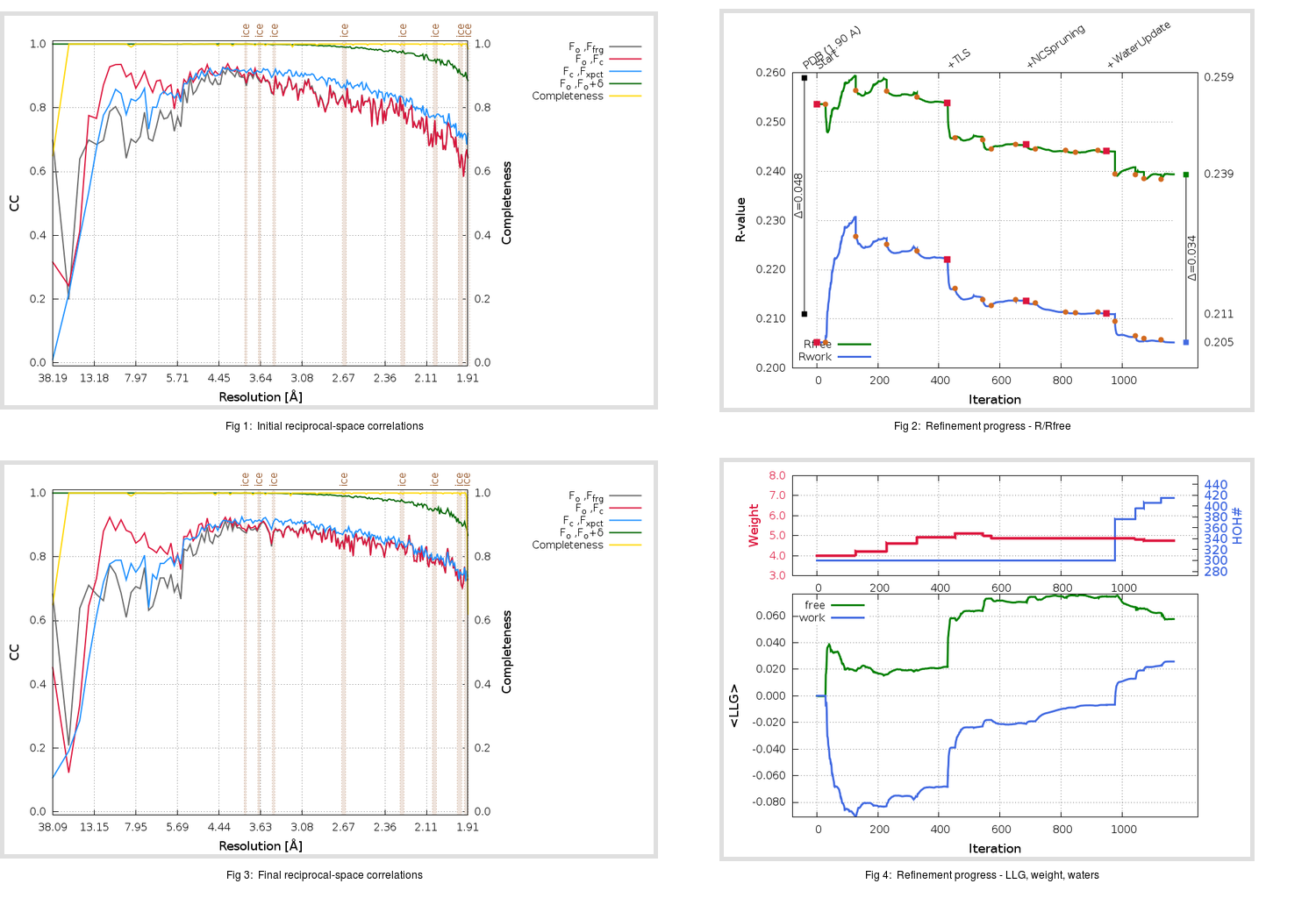Content:

```    Diffraction limits & principal axes of ellipsoid fitted to diffraction cut-off surface:
1.867         0.9865   0.0000   0.1636       0.997 a* - 0.083 c*
1.878         0.0000   1.0000   0.0000       b*
1.875        -0.1636   0.0000   0.9865      -0.127 a* + 0.992 c*
```

## Deposited

` `
 Date deposited Date data collection Resolution R, Rfree 20030725 20030101 1.90 0.2110 0.2590

Molprobity (CCP4 7.0 version) summary:

```Ramachandran outliers =   1.50 %
favored =  94.49 %
Rotamer outliers      =   6.36 %
C-beta deviations     =     0
Clashscore            =  19.66
RMS(bonds)            =   0.0133
RMS(angles)           =   1.77
MolProbity score      =   2.78
Resolution            =   1.90
R-work                =   0.2110
R-free                =   0.2590
```

```Number of waters      =   300

<B> (all atoms) =   28.66 ( sd =   15.23 ) for       4975 non-hydrogen atoms
<B>   (protein) =   28.33 ( sd =   15.49 ) for       4675 non-hydrogen atoms
<B>     (water) =   33.79 ( sd =    8.98 ) for        300 non-hydrogen atoms
<B>    (others) =    0.00 ( sd =    0.00 ) for          0 non-hydrogen atoms

B min/max       (all non-hydrogen atoms) =    7.54 /  113.80
B min/max   (protein non-hydrogen atoms) =    7.54 /  113.80
B min/max     (water non-hydrogen atoms) =   12.38 /   51.05
B min/max     (other non-hydrogen atoms) =    0.00 /    0.00
```

## BUSTER (re-)refinement

` `

Molprobity (CCP4 7.0 version) summary:

```Ramachandran outliers =   0.00 %
favored =  97.33 %
Rotamer outliers      =   4.62 %
C-beta deviations     =     8
Clashscore            =   5.40
RMS(bonds)            =   0.0114
RMS(angles)           =   1.60
MolProbity score      =   1.92
Resolution            =   1.91
R-work                =   0.2052
R-free                =   0.2394
```

```Number of waters      =   415

<B> (all atoms) =   27.40 ( sd =   11.59 ) for       5090 non-hydrogen atoms
<B>   (protein) =   26.54 ( sd =   11.13 ) for       4675 non-hydrogen atoms
<B>     (water) =   37.07 ( sd =   12.16 ) for        415 non-hydrogen atoms
<B>    (others) =    0.00 ( sd =    0.00 ) for          0 non-hydrogen atoms

B min/max       (all non-hydrogen atoms) =    9.08 /   79.37
B min/max   (protein non-hydrogen atoms) =    9.08 /   76.43
B min/max     (water non-hydrogen atoms) =   13.67 /   79.37
B min/max     (other non-hydrogen atoms) =    0.00 /    0.00
```

Refinement progression:Results:

` `
 File Remark 1UJ1_aB_refine.01_04_refine.pdb.gz exact refinement commands are in header 1UJ1_aB_refine.01_04_refine.mtz.gz including original deposited data and several re-refinement map coefficients 1UJ1_aB_refine.01_04_BUSTER_model.cif.gz including any non-standard compound restraints 1UJ1_aB_refine.01_04_BUSTER_refln.cif.gz